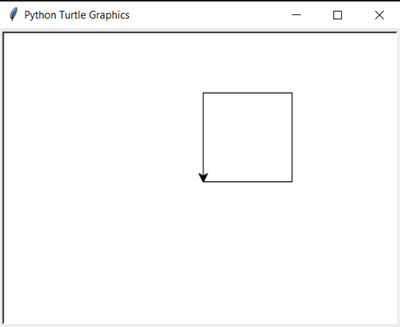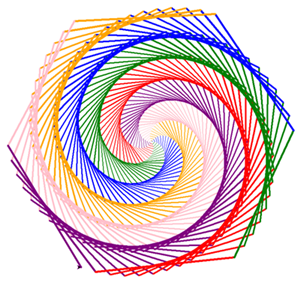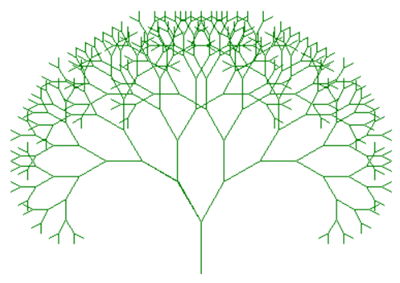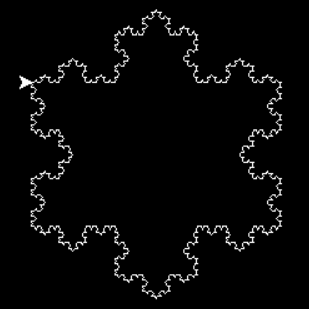# Teaching kids how to code with Python Turtle

Teaching how to code from a young age has become increasingly usual. There are good reasons to do so, since the ability to code has been viewed as one of the most important for the years to come, but also — and probably more importantly — because learning to code offers kids a way to develop and train a range of different skills perceived as useful in everyday life and in other school subjects, like creativity, problem solving, persistence and even collaboration.

Although learning how to code can be difficult and scary, there are some approaches that can mitigate those difficulties. First of all, we should — obviously — take the age of the kid into consideration, but also have in mind that learning should be fun.

«El cerebro sólo aprende si hay emoción» (The brain only learns if there is emotion)

Francisco Mora, neuroeducation specialist

I was about 15 or 16 when I started programming and I did it using BASIC (Beginner’s All-purpose Symbolic Instruction Code). But there’s a chance that some of you reading this text first learned programming — or at least are familiar — with the Logo language, especially if you are a little older than me.

Logo is known by its turtle graphics that are vector graphics / images produced by a small turtle-shaped cursor moving around the screen, obeying to a list of programmed instructions. It requires some geometry knowledge, particularly on angles and angle measure. That means that — in theory — kids around 8-10 years old should be able to understand the mechanics of the exercises to be performed.

The disadvantage of learning Logo is that it has little to no practical use in the real world. It was conceived only to teach programming concepts and is a somewhat dead language.

Fortunately, Python has a turtle module that can be used to learn coding the same way you would with Logo, although — obviously — using a different syntax.

Python is the most widely used programming language worldwide and the one that has grown the most in recent years. And it is easy to explain why. It’s easy to read and to learn; it is very versatile, with its uses ranging from web development to data science and artificial intelligence, not forgetting its ease of use to write simple scripts that can automate many of your day-to-day tasks; it’s also highly extensible with its library system. Python is used not only by programmers, but also by many researchers, students and amateur coders, which helps to explain its growth and position as market leader.

# How to use Python turtle?

So, the idea is to command the cursor to move around, starting on the (0, 0) position of the x-y cartesian plane. Once imported, the turtle module has instructions to move the cursor, like «forward(20)» — to move it 20 pixels in the direction it is facing — or «right(90)» — to rotate 90 degrees clockwise. Using only these simple commands, the cursor can draw shapes and pictures the way you want.

Easy, right?

The code below instructs the drawing of a square.

`import turtleturtle.forward(20)turtle.left(90)turtle.forward(20)turtle.left(90)turtle.forward(20)turtle.left(90)turtle.forward(20)turtle.done()`As you probably noticed, the default shape of the cursor is not a turtle, as on Logo, but an arrow. But that can be changed with the instruction «turtle.shape(‘turtle’)».

The code above uses the object oriented programming paradigm. Although in a simple way (just a turtle object with three methods: forward, left and done), it can still be a difficult concept to explain to those who are starting to program. If we want to simplify, we can work around this eventual problem using another technique for importing the turtle package.

`from turtle import *forward(20)left(90)forward(20)left(90)forward(20)left(90)forward(20)done()`

Although it is not a recommended practice on «real» programming, in this case it makes the code more easily readable and helps to keep the focus on what ‘s important, without the need to explain — as such an early stage — the concepts of object and object-oriented language.

# What can we draw with Python turtle?

Well, basically everything, if you have the skills. But we should start with the basics, even more when teaching.

## Drawing a Circle

What about starting with a circle? Since the module contains a specific method to draw a circle, we’re going to spice things up a little by changing the line colour. The following code draws a 50-pixels radius circle. Well, if we’re strict, it’s actually a circumference of a circle.

`# import modulefrom turtle import *# set line color to redcolor('red')# draw a circle with radius of 50 pixelscircle(50)# end the drawingdone()`

To fill the area inside the circumference we just have to add three more instructions.

`# import modulefrom turtle import *# set line color to red and fill color to orangecolor('red')fillcolor('orange')begin_fill()# draw a circle with radius of 50 pixelscircle(50)end_fill()# end the drawingdone()`

## Drawing polygons

Let’s start by drawing a hexagon.

`from turtle import *for i in range(6):  forward(100)  left(60)done()`

It’s the right time to introduce the students to loops, particularly a for loop. Since the Python method «range(n)» creates a sequence of n numbers (ranging from 0 to n-1), the line «for i in range(6)» will instruct Python to execute the code inside the for loop for 6 times. That means that the cursor will go forward 100 pixels, then turn left 60º and repeat these two steps for a total of 6 times.

Why 60º? Well, because it’s the result of dividing 360º by 6 (the number of sides of the polygon).

As you can see, learning this way is not only about learning to code; it also helps students to better understand geometry and train geospatial comprehension skills.

Generalizing the code above, we can redefine it to create any polygon, given the desired number of sides.

The one showed below will draw a 5-sides polygon — a pentagon.

`from turtle import *number_of_sides = 5for i in range(number_of_sides):  forward(100)  left(360 / number_of_sides)done()`

The main difference lies in the use of a variable to save the desired number of sides. Probably, kids in the age range mentioned earlier (8–10 years) are not yet familiarized with the mathematical concept of variable. But it shouldn’t be hard to explain them that it only represents a mean to store a value that can be changed.

## The sky is the limit

Knowing the basics and with access to the package’s full reference, there are many activities and challenges that can be devised and put into practice.

I leave some examples below.

First, a rainbow benzene with six different colours.

`from turtle import *colours = ['red', 'green', 'blue', 'orange', 'pink', 'purple']speed(10)for x in range(360):  pencolor(colours[x % 6])  width(x / 100 + 1)  forward(x)  left(59)`The difference of one degree (in relation to the 60 used to draw a hexagon) slightly tilts the drawing, creating this visual effect.

The used angle of turn of 59º is meant to add a slight variation factor from the hexagon shape. The new methods used should be easy to understand. The «speed» method allows to change the drawing speed. The «pencolor» method changes the colour of the pen.

Now, a green-branched fractal tree.

`import turtlet = turtle.Turtle()t.color('green')def draw_tree(t, branch_length, branch_shortening_factor, angle):  if branch_length > 5:    t.forward(branch_length)    new_branch_length = branch_length - branch_shortening_factort.left(angle)    draw_tree(t, new_branch_length, branch_shortening_factor, angle)t.right(angle * 2)    draw_tree(t, new_branch_length, branch_shortening_factor, angle)t.left(angle)    t.backward(branch_length)draw_tree(t, 50, 5, 30)turtle.mainloop()`The tree is drawn recursively, in a fractal way.

And finally, a Koch Snowflake.

`import turtlet = turtle.Turtle()wn = turtle.Screen()wn.bgcolor('black')t.color("white")t.speed(0)def koch(cursor, iterations, length, shortening_factor, angle):    if iterations == 0:        cursor.forward(length)    else:        iterations = iterations - 1        length = length / shortening_factor            koch(cursor, iterations, length, shortening_factor, angle)        cursor.left(angle)        koch(cursor, iterations, length, shortening_factor, angle)        cursor.right(angle * 2)        koch(cursor, iterations, length, shortening_factor, angle)        cursor.left(angle)        koch(cursor, iterations, length, shortening_factor, angle)for i in range(3):    koch(t, 4, 200, 3, 60)    t.right(120)    turtle.mainloop()`The Koch Snowflake is a fractal curve presented by Swedish mathematician Helge von Koch.

The construction of the two figures above is— obviously — way too complex to be understood by 8–10 year old kids. But can be a good way to explain concepts like recursion to older students.

Full reference on Python’s turtle module can be found here: https://docs.python.org/3/library/turtle.html.

--

--